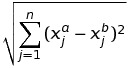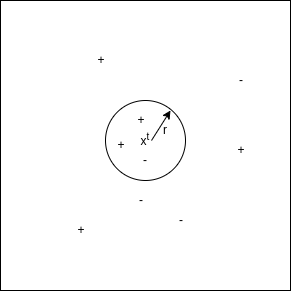# Implementation of Radius Neighbors from Scratch in Python

• Last Updated : 27 Sep, 2021

Radius Neighbors is also one of the techniques based on instance-based learning. Models based on instance-based learning generalize beyond the training examples. To do so, they store the training examples first. When it encounters a new instance (or test example), then they instantly build a relationship between stored training examples and this new instant to assign a target function value for this new instance. Instance-based methods are sometimes called lazy learning methods because they postponed learning until the new instance is encountered for prediction.

Instead of estimating the hypothetical function (or target function) once for the entire space,  these methods will estimate it locally and differently for each new instance to be predicted.

#### Radius Neighbors Classifier:

Basic Assumptions

1. All instances correspond to points in the n-dimensional space where n represents the number of features in any instance.
2. The neighbors of an instance are defined in terms of the Euclidean distance.
An instance can be represented by < x1, x2, .............., xn >.
Euclidean distance between two instances xa and xb is given by d( xa, xb ) :Euclidean Distance

How does it work?

Radius Neighbors Classifier first stores the training examples. During prediction, when it encounters a new instance ( or test example) to predict, it finds the number of neighbors from training instances within a fixed radius of r center at test instance, where r is a floating-point value specified by the user.  Then assigns the most common class among the training instances within that radius to the test instance.

The optimal choice for r is by validating errors on test data.Radius Neighbors Classification Graphical Representation

In the above figure, “+” denotes training instances labelled with 1. “-” denotes training instances with 0. Here we classified for the test instance xt as the most common class among training instances within the circle. Here, r is a user-specified choice. In the above figure, positive instances are in majority in the circle, so xt is classified as “+” or 1.

#### Pseudocode:

1. Store all training instances.
2. Repeat steps 3, 4, and 5 for each test instance.
3. Finds the number of neighbors from training instances within a fixed radius of r center at a test instance.
4. y_pred for current test example =  most common class among training instances within a circle.
5. Go to step 2.

#### Implementation

Diabetes Dataset used in this implementation can be downloaded from link.

It has 8 features columns like i.e “Age”, “Glucose” e.t.c, and the target variable “Outcome” for 108 patients. So in this, we will create a K-Nearest Neighbors Classifier model to predict the presence of diabetes or not for patients with such information.

## Python3

 # Importing libraries import pandas as pd import numpy as np from sklearn.model_selection import train_test_split from scipy.stats import mode from sklearn.neighbors import RadiusNeighborsClassifier # Radius Nearest Neighbors Classification class Radius_Nearest_Neighbors_Classifier() :         def __init__( self, r ) :                 self.r = r             # Function to store training set             def fit( self, X_train, Y_train ) :                 self.X_train = X_train                 self.Y_train = Y_train                 # no_of_training_examples, no_of_features                 self.m, self.n = X_train.shape         # Function for prediction             def predict( self, X_test ) :                 self.X_test = X_test                 # no_of_test_examples, no_of_features                 self.m_test, self.n = X_test.shape                 # initialize Y_predict                 Y_predict = np.zeros( self.m_test )                 for i in range( self.m_test ) :                         x = self.X_test[i]                         # find the number of neighbors within a fixed            # radius r of current training example                         neighbors = self.find_neighbors( x )                         # most frequent class in the circle drawn by current            # training example of fixed radius r                         Y_predict[i] = mode( neighbors )                     return Y_predict         # Function to find the number of neighbors within a fixed radius    # r of current training example               def find_neighbors( self, x ) :                 # list to store training examples which will fall in the circle                 inside = []                 for i in range( self.m ) :                         d = self.euclidean( x, self.X_train[i] )                         if d <= self.r :                                 inside.append( self.Y_train[i] )         inside_array = np.array( inside )                         return inside_array         # Function to calculate euclidean distance                 def euclidean( self, x, x_train ) :                 return np.sqrt( np.sum( np.square( x - x_train ) ) )  # driver code def main() :         # Create dataset         df = pd.read_csv( "diabetes.csv" )     X = df.iloc[:,:-1].values     Y = df.iloc[:,-1:].values         # Splitting dataset into train and test set     X_train, X_test, Y_train, Y_test = train_test_split(      X, Y, test_size = 1/3, random_state = 0 )         # Model training         model = Radius_Nearest_Neighbors_Classifier( r = 550 )         model.fit( X_train, Y_train )         model1 = RadiusNeighborsClassifier( radius = 550 )         model1.fit( X_train, Y_train )         # Prediction on test set     Y_pred = model.predict( X_test )         Y_pred1 = model1.predict( X_test )         # measure performance         correctly_classified = 0         correctly_classified1 = 0         # counter         count = 0         for count in range( np.size( Y_pred ) ) :                 if Y_test[count] == Y_pred[count] :                         correctly_classified = correctly_classified + 1                     if Y_test[count] == Y_pred1[count] :                         correctly_classified1 = correctly_classified1 + 1                 count = count + 1             print("Accuracy on test set by our model     : ", (      correctly_classified / count ) * 100 )         print("Accuracy on test set by sklearn model : ", (      correctly_classified / count ) * 100 )   if __name__ == "__main__" :         main()

#### Output  :

Accuracy on test set by our model     :  61.111111111111114
Accuracy on test set by sklearn model :  61.111111111111114

The accuracy achieved by our model and sklearn is equal which indicates the correct implementation of our model.

Note: Above Implementation is for model creation from scratch, not to improve the accuracy of the diabetes dataset.

#### Radius Neighbors Regressor:

Radius Neighbors Regressor first stores the training examples. During prediction, when it encounters a new instance ( or test example ) to predict,  it finds the number of neighbors from training instances within a fixed radius of r center at test instance, where r is a floating-point value specified by the user.  Then assigns the mean of the training instances within that radius to the test instance.

The optimal choice for r is by validating errors on test data.

#### Pseudocode:

1. Store all training instances.
2. Repeat steps 3, 4, and 5 for each test instance.
3. Finds the number of neighbors from training instances within a fixed radius of r center at a test instance.
4. y_pred for current test example =  mean of the training instances within a circle.
5. Go to step 2.

#### Implementation:

Dataset used in this implementation can be downloaded from link.

It has 2 columns — “YearsExperience” and “Salary” for 30 employees in a company. So in this, we will create a Radius Neighbors Regression model to learn the correlation between the number of years of experience of each employee and their respective salary.

The model, we created predicts the same value as the sklearn model predicts for the test set.

Code:

## Python3

 # Importing libraries import pandas as pdimport numpy as npfrom sklearn.model_selection import train_test_splitfrom sklearn.neighbors import RadiusNeighborsRegressor # Radius Nearest Neighbors Regression class Radius_Nearest_Neighbors_Regression() :         def __init__( self, r ) :                 self.r = r             # Function to store training set             def fit( self, X_train, Y_train ) :                 self.X_train = X_train                 self.Y_train = Y_train                 # no_of_training_examples, no_of_features                 self.m, self.n = X_train.shape         # Function for prediction             def predict( self, X_test ) :                 self.X_test = X_test                 # no_of_test_examples, no_of_features                 self.m_test, self.n = X_test.shape                 # initialize Y_predict                 Y_predict = np.zeros( self.m_test )                 for i in range( self.m_test ) :                         x = self.X_test[i]                         # find the number of neighbors within a fixed            # radius r of current training example                         neighbors = self.find_neighbors( x )                         # mean of the neighbors in the circle drawn by            # current training example of fixed radius r                         Y_predict[i] = np.mean( neighbors )                     return Y_predict         # Function to find the number of neighbors within a fixed    # radius r of current training example               def find_neighbors( self, x ) :                 # list to store training examples which will fall in the circle                 inside = []                 for i in range( self.m ) :                         d = self.euclidean( x, self.X_train[i] )                         if d <= self.r :                                 inside.append( self.Y_train[i] )         inside_array = np.array( inside )                         return inside_array         # Function to calculate euclidean distance                 def euclidean( self, x, x_train ) :                 return np.sqrt( np.sum( np.square( x - x_train ) ) )        # driver code def main() :         # Importing dataset         df = pd.read_csv( "salary_data.csv" )     X = df.iloc[:,:-1].values     Y = df.iloc[:,1].values         # Splitting dataset into train and test set     X_train, X_test, Y_train, Y_test = train_test_split(      X, Y, test_size = 1/3, random_state = 0 )         # Model training         model = Radius_Nearest_Neighbors_Regression( r = 550 )         model.fit( X_train, Y_train )         model1 = RadiusNeighborsRegressor( radius = 550 )     model1.fit( X_train, Y_train )         # Prediction on test set         Y_pred = model.predict( X_test )     Y_pred1 = model1.predict( X_test )         print( "Real values                         : ", Y_test[:3] )         print( "Predicted values by our model       : ", np.round( Y_pred[:3], 2 ) )         print( "Predicted values by sklearn model   : ", np.round( Y_pred1[:3], 2 ) )     if __name__ == "__main__" :         main()

Output:

Real values                         :  [ 37731 122391  57081]
Predicted values by our model       :  [71022.5 71022.5 71022.5]
Predicted values by sklearn model   :  [71022.5 71022.5 71022.5]

My Personal Notes arrow_drop_up